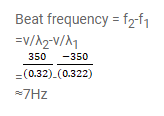# Calculate the frequency of beats producedQuestion:

Calculate the frequency of beats produced in air when two sources of sound are activated, one emitting a wavelength of 32 $\mathrm{cm}$ and the other of $32.2 \mathrm{~cm}$. The speed of sound in air is $350 \mathrm{~m} \mathrm{~s}^{-1}$.

Solution: